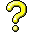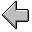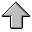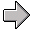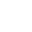Functional Programming4. Techniques for Functional Programming

## 4.1 Abstract, Map, Fold/Reduce, Recurse!

We now begin looking at various idioms for functional programming. The basic strategy of all programming is of course:

• Decompose - break the problem into smaller pieces.

• Abstract - identify the operations you need and build small functions and data structures to perform them.
Once you have broken your problem into smaller pieces, the following general guidelines hold for approaching the problem in a functional way:

1. If the problem can be expressed as a bunch of independent parallel subproblems, then consider `map`.

2. If the problem can be expressed as a traversal over the data while accumulating the final result, consider `fold/reduce`.

3. If the problem can be expressed in terms of smaller instances of the same problem, then consider recursion.
The general idea is to not implement something when an existing operator will do. For example, don't write these:
code/LambdaScheme/tech-01.rkt

 `    ``#lang racket` `    `` ` `    ``(define T '(1 3 5 7 9))` `    `` ` `    ``; Eg 1 - hand-crafted map of add 1` `    `` ` `    ``(define (add1-to-list L) ` `    ``    (if (null? L) '() (cons (+ 1 (first L)) (add1-to-list (rest L)))) )` `    `` ` `    ``(add1-to-list T)` `    `` ` `    ``; Eg 2 - hand-crafted fold/reduce of +` `    `` ` `    ``(define (sum-list L)` `    ``    (if (null? L) 0` `    ``        (+ (first L) (sum-list (rest L) ) ) ) )` `    `` ` `    ``(sum-list T)` `    `` `
when these will do
code/LambdaScheme/tech-02.rkt

 `    ``#lang racket` `    `` ` `    ``(define T '(1 3 5 7 9))` `    `` ` `    ``; Eg 1 - use map operator on your own add 1 function` `    `` ` `    ``(map (lambda(x) (+ 1 x)) T)` `    `` ` `    ``; or even better, use the built in function` `    ``(map add1 T)` `    `` ` `    `` ` `    ``; Eg 2 - use the fold operator` `    `` ` `    ``(foldl (lambda (x r) (+ x r)) 0 T)` `    `` ` `    ``; or even better for most algebraic style operations that take arbitrary ` `    ``; numbers of parameters, apply will pass the whole list and the operator` `    ``; will do the folding.` `    `` ` `    ``(apply + T)` `    `` `

## 4.2 Compute Once, Reuse Many

code/LambdaScheme/ComputeAndReuse.rkt

 `    ``#lang racket` `    `` ` `    ``; Often it makes sense to only evaluate a term once and` `    ``; then use that value where needed` `    `` ` `    ``(+ (* 3 4) (- (* 3 4) (/ 1 (* 3 4))))` `    `` ` `    ``; replace each (* 3 4) by variable p which is pre-computed` `    ``(define p (* 3 4))` `    ``(+ p (- p (/ 1 p)))` `    `` ` `    ``; wrap the expression in a closure that captures the p` `    ``; and apply it to the result of computing the term` `    ``( (lambda (p) (+ p (- p (/ 1 p))) )` `    ``  (* 3 4)` `    ``  )` `    `` ` `    ``; you can do this as much as you want` `    ``( (lambda (t1 t2 t3)` `    ``    (if (< t1 t2) (* t1 t3) (* t2 t3))` `    ``    )` `    ``  ` `    ``  (+ 1 3) ` `    ``  (- 4 6)` `    ``  7` `    ``  )` `    `` ` `    `` ` `    ``; in unrestricted Scheme, use the let construction, which places` `    ``; the computation of the value beside the variable it is assigned to` `    ``(let ( (p (* 3 4)) )` `    ``  (+ p (- p (/ 1 p)))` `    ``  )` `    `` ` `    ``(let (` `    ``      (t1 (+ 1 3))` `    ``      (t2 (- 4 6))` `    ``      (t3 7)` `    ``      )` `    ``  (if (< t1 t2) (* t1 t3) (* t2 t3))` `    ``  )`
Fiannly, first correctness deal with correctness, then worry about efficiency! If compute time or space is an issue, consider how to restructure the algorithm, say to make it tail-recrusive, or reduce redundant computation.

## 4.3 Use `cond` instead of `if` for guarded commands

There a two main styles of conditional processing. Nested `if` statements typically reflect a decision-tree style of algorithm. The logic can become quite deep and impenetrable. The other style of logic is the guarded command composed of a sequence of commands where each command is guarded by a conditional. The first command with a true guard is executed. The `cond` statement is a guarded command that returns the value of the expression that was evaluated.

An example of this issue is illustrated in the section below on restructuring code for clarity.

## 4.4 Pass State Down the Recursion

code/LambdaScheme/MaxIndex.rkt

 `    ``#lang racket` `    `` ` `    ``; find position of max in a list` `    ``; helper function simply passes along all the state that is needed for` `    ``; the computation.` `    `` ` `    ``; note the tail recursion, so the stack is never more than constant size` `    `` ` `    ``; Pre: L is a non-empty list of numbers` `    ``; Post: returns position of first maximum in list, 0-origin indexing` `    ``(define lmax-index` `    ``  (lambda (L)` `    ``    (lmax-index-r (first L) 0 1 (rest L) )` `    ``    )` `    ``  )` `    `` ` `    ``; lmax-index recursor` `    ``; cur-max - value of the current maximum` `    ``; cur-index - position of the current maximum` `    ``; L-index - position of the first element of the remaining list` `    ``; L - remaining list of numbers to be processed` `    `` ` `    ``(define lmax-index-r ` `    ``  ( lambda (cur-max cur-index L-index L)` `    ``     (if (null? L) ` `    ``         cur-index` `    ``         (if (>= cur-max (first L))` `    ``             ; max still good (it is at least as big as the first element)` `    ``             (lmax-index-r cur-max cur-index (+ 1 L-index) (rest L))` `    ``             ; first is new max` `    ``             (lmax-index-r (first L) L-index (+ 1 L-index) (rest L))` `    ``             )` `    ``         )` `    ``     )` `    ``  )` `    `` ` `    ``(lmax-index '(1 2 3 4))` `    ``; NOTE: what happens if the >= test above is changed to a > test?` `    ``  ` `    ``; Now create a function that returns a list of the positions of ` `    ``; all the maximums in L.` `    ``; This would work for an empty list, since the list of positions` `    ``; would be empty`

## 4.5 Use Local Functions to Avoid Passing Common Data

When you define a function inside the scope of another function, the definition captures the current values of any variables. Foe example

code/LambdaScheme/Closure.rkt

 `    ``#lang racket` `    `` ` `    ``; an example of how a lambda term is a closure that captures its local` `    ``; environment` `    `` ` `    ``; the inner lambda captures the value of x on construction of the closure.` `    ``(define f-of-1 (lambda (x)` `    ``    (lambda (y) (+ x y))) )` `    `` ` `    ``(define inc-by-1 (f-of-1 1))` `    ``(define inc-by-42 (f-of-1 42))` `    `` ` `    ``(inc-by-42 1)` `    ``(inc-by-1 3)`

## 4.6 Restructure Your Code for Clarity

The judicious use of `let` and inner functions results in considerably simpler code. In this sequence of examples we beging with ugly code that has nested `if` statements that are really guarded commands, we are dragging the original function arguments down the recursion, and we are making multiple recursion calls that all look very similar.

code/LambdaScheme/fmap2/fmap2lcm-ugly.rkt

 `    ``#lang racket` `    `` ` `    `` ` `    ``; If X = (x_0 x_1 ... x_n) and Y = (y_0 y_1 ... y_m) then` `    ``;       (fmap2lcm fn X Y)` `    ``; is the list (r_0 r_1 ... r_l) where where the element r_i` `    ``; in position i is (fn x_(i mod n) y_(i mod m))` `    ``; and l = lcm(m, n) where lcm - least common multiple.` `    ``;` `    ``; In other words the map is performed by reusing the lists X and Y until` `    ``; they both end at the same time.` `    `` ` `    ``; Example: (fmap2lcm list '(1 2) '(3 4 5)) is ` `    ``; ((1 3) (2 4) (1 5) (2 3) (1 4) (2 5))` `    `` ` `    ``; This is a very ugly implementation` `    ``; We have a seriously nested set of if-statments, and are dragging the` `    ``; original input lists through the recursion.` `    `` ` `    ``(define fmap2lcm-r` `    ``  (lambda (fn X-cur Y-cur X-orig Y-orig)` `    ``    (if (and (null? X-cur) (null? Y-cur))` `    ``        '()` `    ``        (if (null? X-cur)` `    ``            (fmap2lcm-r fn X-orig Y-cur X-orig Y-orig)` `    ``            (if (null? Y-cur)` `    ``                (fmap2lcm-r fn X-cur Y-orig X-orig Y-orig)` `    ``                (cons (fn (first X-cur) (first Y-cur))` `    ``                      (fmap2lcm-r fn (rest X-cur) (rest Y-cur) X-orig Y-orig)` `    ``                )` `    ``            )))))` `    `` ` `    ``(define fmap2lcm` `    ``  (lambda (fn X Y)` `    ``    ; handle special boundary case of empty input lists` `    ``    (if (or (null? X) (null? Y)) '() (fmap2lcm-r fn X Y X Y)))`

code/LambdaScheme/fmap2/fmap2lcm-clean.rkt

 `    ``#lang racket` `    `` ` `    `` ` `    ``; If X = (x_0 x_1 ... x_n) and Y = (y_0 y_1 ... y_m) then` `    ``;       (fmap2lcm fn X Y)` `    ``; is the list (r_0 r_1 ... r_l) where where the element r_i` `    ``; in position i is (fn x_(i mod n) y_(i mod m))` `    ``; and l = lcm(m, n) where lcm - least common multiple.` `    ``;` `    ``; In other words the map is performed by reusing the lists X and Y until` `    ``; they both end at the same time.` `    `` ` `    ``; Example: (fmap2lcm list '(1 2) '(3 4 5)) is ` `    ``; ((1 3) (2 4) (1 5) (2 3) (1 4) (2 5))` `    `` ` `    ``; Cleanup:` `    ``; Since the agorithm is more a sequence of guarded commands instead of a` `    ``; decision tree, we replace the nested if-statements with a cond-statement` `    ``; ` `    `` ` `    ``(define fmap2lcm-r` `    ``  (lambda (fn X-cur Y-cur X-orig Y-orig)` `    ``    (cond ` `    ``     ; both lists done at same time - have reached lcm` `    ``     [ (and (null? X-cur) (null? Y-cur)) '() ]` `    `` ` `    ``     ; X runs out first, recycle` `    ``     [ (null? X-cur) (fmap2lcm-r fn X-orig Y-cur X-orig Y-orig) ]` `    `` ` `    ``     ; Y runs out first, recycle` `    ``     [ (null? Y-cur) (fmap2lcm-r fn X-cur Y-orig X-orig Y-orig) ]` `    `` ` `    ``     ; process first of both and recurse.` `    ``     [ else (cons ` `    ``                (fn (first X-cur) (first Y-cur))` `    ``                (fmap2lcm-r fn (rest X-cur) (rest Y-cur) X-orig Y-orig)) ]` `    ``    )))` `    `` ` `    ``; We are still dragging the original input lists through the recursion.` `    `` ` `    ``(define fmap2lcm` `    ``  (lambda (fn X Y)` `    ``    ; handle special boundary case of empty input lists` `    ``    (if (or (null? X) (null? Y)) '() (fmap2lcm-r fn X Y X Y))))`

code/LambdaScheme/fmap2/fmap2lcm-cleaner.rkt

 `    ``#lang racket` `    `` ` `    ``; If X = (x_0 x_1 ... x_n) and Y = (y_0 y_1 ... y_m) then` `    ``;       (fmap2lcm fn X Y)` `    ``; is the list (r_0 r_1 ... r_l) where where the element r_i` `    ``; in position i is (fn x_(i mod n) y_(i mod m))` `    ``; and l = lcm(m, n) where lcm - least common multiple.` `    ``;` `    ``; In other words the map is performed by reusing the lists X and Y until` `    ``; they both end at the same time.` `    `` ` `    ``; Example: (fmap2lcm list '(1 2) '(3 4 5)) is ` `    ``; ((1 3) (2 4) (1 5) (2 3) (1 4) (2 5))` `    `` ` `    ``; Cleanup:` `    ``; We move the definition of the recursor function fmap2lcm-r inside the main` `    ``; function.  This means that the recursor has access to the original X and Y` `    ``; lists, so they do not have to be passed down the recursion.` `    `` ` `    ``(define fmap2lcm` `    ``  (lambda (fn X Y)` `    ``    ` `    ``    (define fmap2lcm-r` `    ``      (lambda (fn X-cur Y-cur)` `    ``        (cond ` `    ``         ; both lists done at same time - have reached lcm` `    ``         [ (and (null? X-cur) (null? Y-cur)) '() ]` `    `` ` `    ``         ; X runs out first, recycle` `    ``         [ (null? X-cur) (fmap2lcm-r fn X Y-cur) ]` `    `` ` `    ``         ; Y runs out first, recycle` `    ``         [ (null? Y-cur) (fmap2lcm-r fn X-cur Y) ]` `    `` ` `    ``         ; process first of both and recurse.` `    ``         [ else (cons ` `    ``                    (fn (first X-cur) (first Y-cur))` `    ``                    (fmap2lcm-r fn (rest X-cur) (rest Y-cur) )) ]` `    ``        )))` `    `` ` `    ``    ; handle special boundary case of empty input lists` `    ``    (if (or (null? X) (null? Y)) '() (fmap2lcm-r fn X Y))` `    ``))`

code/LambdaScheme/fmap2/fmap2lcm-cleanest.rkt

 `    ``#lang racket` `    `` ` `    ``; If X = (x_0 x_1 ... x_n) and Y = (y_0 y_1 ... y_m) then` `    ``;       (fmap2lcm fn X Y)` `    ``; is the list (r_0 r_1 ... r_l) where where the element r_i` `    ``; in position i is (fn x_(i mod n) y_(i mod m))` `    ``; and l = lcm(m, n) where lcm - least common multiple.` `    ``;` `    ``; In other words the map is performed by reusing the lists X and Y until` `    ``; they both end at the same time.` `    `` ` `    ``; Example: (fmap2lcm list '(1 2) '(3 4 5)) is ` `    ``; ((1 3) (2 4) (1 5) (2 3) (1 4) (2 5))` `    `` ` `    ``; Cleanup:` `    ``; we make a final cleanup by observing that we can have just one recursive ` `    ``; call point if we reset the list that ran out.  Then we can eliminate the` `    ``; multiple cases in the cond and restore the if.` `    `` ` `    ``(define fmap2lcm` `    ``  (lambda (fn X Y)` `    ``    ` `    ``    ; recursor ` `    ``    (define fmap2lcm-r` `    ``      (lambda (fn X-cur Y-cur)` `    ``         ( let (` `    ``                ; wrap X-cur and Y-cur if they are finished` `    ``                [ X-r (if (null? X-cur) X X-cur) ]` `    ``                [ Y-r (if (null? Y-cur) Y Y-cur) ]` `    ``                )` `    `` ` `    ``            (if (and (null? X-cur) (null? Y-cur)) ` `    ``                ; when both empty have hit the lcm` `    ``                '() ` `    `` ` `    ``                ; otherwise process one more element and recurse` `    ``                (cons ` `    ``                    (fn (first X-r) (first Y-r))` `    ``                    (fmap2lcm-r fn (rest X-r) (rest Y-r) ))` `    ``                ) ` `    ``        ))) ; end of fmap2lcm-r` `    `` ` `    ``    ; handle special boundary case of empty input lists` `    ``    (if (or (null? X) (null? Y)) '() (fmap2lcm-r fn X Y))` `    ``))` `    `` `

## 4.7 Use an Accumulator to Pass Partial Results Down

The idea of an accumulator is to save the result immediately rather than waiting for the recursion to return. One unstated advantage of using accumulators is that during debugging the accumulator holds all the results processed so far.

code/LambdaScheme/Reverse.rkt

 `    ``#lang racket` `    `` ` `    ``; tail recursion is very cheap, structure your code to use it when` `    ``; possible.  You may have to introduce a helper function` `    `` ` `    ``; notice how the stack never gets deeper than two calls` `    `` ` `    ``(define lreverse-acc` `    ``  (lambda (L) (lreverse-r L '()))` `    ``  )` `    `` ` `    ``; the recursor helper function accumulates the final result in out-list` `    ``; out-list is called an accumulator` `    `` ` `    ``; notice how the stack never gets deeper than two calls` `    `` ` `    ``(define lreverse-r ` `    ``  (lambda (in-list out-list)` `    ``    (if (null? in-list) ` `    ``        out-list` `    ``        (lreverse-r (rest in-list) (cons (first in-list) out-list))` `    ``        )` `    ``    )` `    ``  )` `    `` ` `    ``(lreverse-r '() '())` `    ``(lreverse-r '(1) '())` `    ``(lreverse-r '(1 2 3 4) '())` `    ``(lreverse-acc '(1 2 3 4)) `

This technique can be used for the `fmap2lcm` function above.

code/LambdaScheme/fmap2/fmap2lcm-final.rkt

 `    ``#lang racket` `    `` ` `    ``; If X = (x_0 x_1 ... x_n) and Y = (y_0 y_1 ... y_m) then` `    ``;       (fmap2lcm fn X Y)` `    ``; is the list (r_0 r_1 ... r_l) where where the element r_i` `    ``; in position i is (fn x_(i mod n) y_(i mod m))` `    ``; and l = lcm(m, n) where lcm - least common multiple.` `    ``;` `    ``; In other words the map is performed by reusing the lists X and Y until` `    ``; they both end at the same time.` `    `` ` `    ``; Example: (fmap2lcm list '(1 2) '(3 4 5)) is ` `    ``; ((1 3) (2 4) (1 5) (2 3) (1 4) (2 5))` `    `` ` `    ``; Cleanup:` `    ``; This is not so much a cleanup as an optimization to show to use accumulators` `    ``; to introduce tail-recursion` `    `` ` `    ``(define fmap2lcm` `    ``  (lambda (fn X Y)` `    ``    ` `    ``    ; recursor ` `    ``    (define fmap2lcm-r` `    ``      (lambda (fn X-cur Y-cur result)` `    ``         ( let (` `    ``                ; wrap X-cur and Y-cur if they are finished` `    ``                [ X-r (if (null? X-cur) X X-cur) ]` `    ``                [ Y-r (if (null? Y-cur) Y Y-cur) ]` `    ``                )` `    `` ` `    ``            (if (and (null? X-cur) (null? Y-cur)) ` `    ``                ; when both empty have hit the lcm, finish by reversing the` `    ``                ; result accumulator` `    ``                (reverse result)` `    `` ` `    ``                ; otherwise process one more element and recurse` `    ``                (fmap2lcm-r fn (rest X-r) (rest Y-r) ` `    ``                    ; add the element to the accumulator, and then recurse` `    ``                    (cons ` `    ``                        (fn (first X-r) (first Y-r))` `    ``                        result))` `    ``            )` `    `` ` `    ``        )` `    ``        )) ; end of fmap2lcm-r` `    `` ` `    ``    ; handle special boundary case of empty input lists` `    ``    ; result accumulator needs to be reversed` `    ``    (if (or (null? X) (null? Y)) '() ` `    ``        (fmap2lcm-r fn X Y '() ) )` `    ``))` `    `` `

## 4.8 Use Default Parameters to Start the Computation

Often the helper function looks like the main function, except that there are initial values for the accumulator etc. Default parameters can be used to simplify things:

code/LambdaScheme/ReverseDefault.rkt

 `    ``#lang racket` `    `` ` `    ``; using default arguments allows us to eliminate the initialization ` `    ``; function for the recursor.  The [ out-list '() ] parameter means` `    ``; if no out-list is provided, use '() the empty list.` `    `` ` `    ``(define lreverse` `    ``  (lambda (in-list [ out-list '() ] )` `    ``    (if (null? in-list)` `    ``        out-list` `    ``        (lreverse (rest in-list) (cons (first in-list) out-list))` `    ``        )` `    ``    )` `    ``  )` `    `` ` `    ``(lreverse '() '())` `    ``(lreverse '(1) '())` `    ``(lreverse '(1 2 3 4) '())` `    ``(lreverse '(1 2 3 4)) `

## 4.9 Getting the tail back in recursion

code/LambdaScheme/StackOps.rkt

 `    ``#lang racket` `    `` ` `    ``; cons and rest let you push onto and pop off of the head of a list.` `    ``; these are constant time operations.` `    `` ` `    ``; suppose you want to push onto and pop off of the tail of a list` `    ``; what are the time and space requirements for this?` `    `` ` `    ``; get the element at the end of list L if L is non-empty` `    ``; this function uses constant stack space because rtop is` `    ``; in tail recursive position` `    `` ` `    ``(define rtop` `    ``  (lambda (L)` `    ``    (if (null? (rest L))` `    ``        (first L)` `    ``        (rtop (rest L))` `    ``        )` `    ``    )` `    ``  )` `    ``             ` `    ``(rtop '(1 2 3 4))` `    `` ` `    ``; push an element e onto the end of list L` `    ``; this implementation causes the call stack to grow, why?` `    ``; because the result of the recursion is used in constructing` `    ``; the final return value.` `    `` ` `    ``(define rpush ` `    ``  (lambda (L e)` `    ``    (if (null? L) ` `    ``        (cons e '())` `    ``        (cons (first L) (rpush (rest L) e))` `    ``            )` `    ``        )` `    ``    )` `    ``  ` `    `` ` `    ``(rpush '() '())` `    ``(rpush '(()) '())` `    ``(rpush '(1) '())` `    ``(rpush '(1 2 3 4) '())` `    ``(rpush '(1 2 3 4) '(5 6))` `    ``(rpush '(1 2 3 4) 5) ` `    `` ` `    `` ` `    ``                  ` `    ``; pop an element e off the end of list L` `    ``; this implementation causes the call stack to grow, why?` `    ``(define rpop ` `    ``  (lambda (L)` `    ``    (if (null? (rest L)) ` `    ``        '()` `    ``        (cons (first L) (rpop (rest L)))` `    ``        )` `    ``    )` `    ``  )` `    `` ` `    ``(rpop '(1 2 3 4))` `    `` ` `    ``; observing that (rpop L) = (lreverse (rest (lreverse L)))` `    ``; and ` `    ``; that (rpush e L) = (lreverse (cons e (lreverse L)))` `    ``; gives a constant stack size algorithm` `    `` ` `    ``; are there tail recursive versions of rpush and rpop simular to lreverse?`

## 4.10 Prefix Computation

Here is an example of computing using map to transform an entire list and then adding to the beginning.

code/LambdaScheme/Prefix.rkt

 `    ``#lang racket` `    `` ` `    ``; given an associative operator op, and` `    ``; a list (e1 e2 e3 ... en)` `    ``; compute the list` `    ``; e1, e1 op e2, e1 op e2 op e3, ..., e1 op e2 op ... op en ` `    `` ` `    `` ` `    ``; what does this function construct as it recurses?` `    ``; this function is not tail recursive.  Why?` `    `` ` `    ``(define prefix-list ` `    ``  (lambda (op L)` `    ``    (if (null? (rest L))` `    ``        L` `    ``        (cons (first L)` `    ``              (map (lambda (x)(op (first L) x) )` `    ``                   (prefix-list op (rest L))` `    ``                   )` `    ``              )` `    ``        )` `    ``    )` `    ``  )` `    `` ` `    ``;(prefix-list + '(1))` `    ``;(prefix-list + '(1 2 3 4 5))` `    `` `
A less clever and more transparent version using the accumulator-todo idiom, but uses the expensive `append` and `last` operations.

code/LambdaScheme/Prefix1.rkt

 `    ``#lang racket` `    `` ` `    ``(define prefix-list-r` `    ``      (lambda (op L-accum L-todo)` `    ``        (if (null? L-todo)` `    ``            L-accum` `    `` ` `    ``            ; add the next element to the end and recurse` `    ``            (prefix-list-r op` `    ``                ; new L-accum` `    ``                (append L_accum` `    ``                  (list (op (last L-accum) (first L-todo))))` `    ``                (rest L-todo))` `    ``            )` `    ``        )` `    ``      )` `    `` ` `    ``(define prefix-list` `    ``  (lambda (op L)` `    ``    (prefix-list-r op (list (first L)) (rest L))))` `    `` `
And one observes that if we use a reversed accumulator things are much easier.

code/LambdaScheme/Prefix2.rkt

 `    ``#lang racket` `    `` ` `    ``; now accumulate in reverse order, since we keep appending to end anyway.` `    ``; this eliminates the append and last operations` `    `` ` `    ``(define prefix-list-r` `    ``      (lambda (op L-accum L-todo)` `    ``        (if (null? L-todo)` `    ``            ; adjust the result into proper order` `    ``            (reverse L-accum)` `    `` ` `    ``            ; add the next element to the end and recurse` `    ``            (prefix-list-r op` `    ``                ; new L-accum` `    ``                (cons ` `    ``                  (op (first L-accum) (first L-todo))` `    ``                  L-accum)` `    ``                ; shorter todo` `    ``                (rest L-todo))` `    ``            )` `    ``        )` `    ``      )` `    `` ` `    ``(define prefix-list` `    ``  (lambda (op L)` `    ``    (prefix-list-r op (list (first L)) (rest L))))` `    `` `

Inspecting all of the above examples, we see that what is generally going on is that we are walking over a list element by element, accumulating information that is used to compute the final result. In general, this idiom is called folding, and it is so common that there are special operations for this.

There are two kinds of folds: `foldl` which processes the list from left to right, and `foldr` which processes the list from right to left.

The way that fold works is to pass not only the current list element to the processing function, but also the previous result (or an initial value for the first element). Thus
``` > (foldl (lambda (x r) (+ x r)) 0 '(1 2 3)) 6 > (foldl (lambda (x r) (cons (+ x 1) r)) '() '(1 2 3)) '(4 3 2) > (foldr (lambda (x r) (cons (+ x 1) r)) '() '(1 2 3)) '(2 3 4) > (foldr (lambda (x r) (cons (+ x 1) r)) '(0) '(1 2 3)) '(2 3 4 0) ```

We can use `foldl` to perform prefix computation as follows.

code/LambdaScheme/Prefix-fold.rkt

 `    ``#lang racket` `    ``(define (prefix-list op L)` `    ``  ; the prefixes are stacked so need to be reversed to get into` `    ``  ; proper order` `    ``  (reverse (foldl (lambda (e p)` `    ``                    (if (null? p) ` `    ``                        ; base case is single element e from head of` `    ``                        ; list remaining to be processed` `    ``                        (list e)` `    ``                        ; p is a stack of prefixes, most recent on top` `    ``                        ; so e is added on right of top of stack, and` `    ``                        ; then pushed on` `    ``                       ` `    ``                        (cons (op (first p) e) p) ))` `    ``                    ` `    ``                  ; starting case of foldl, list to process` `    ``                    '() L))` `    ``           )` `    `` ` `    ``(prefix-list + '(0 1 2 3 4 5))`4. Techniques for Functional Programming Notes on Functional Programming / Version 2.10 2014-02-24